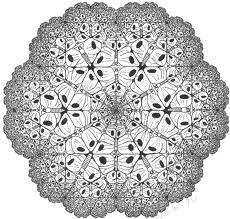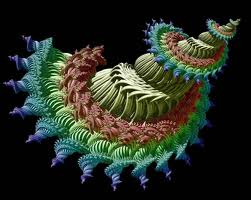### Aim and ScopeAPPLIED MATHEMATICS AND MODELING: Computational Methods, Ordinary and partial Differential Equations, Mathematical Modeling and Optimization, Probability and Statistics Applications, Operations research, model selection, Bio Mathematics, Data Analysis and related topics.  Mathematical Finance, Numerical Solution of Stochastic Differential Equations, Stochastic Analysis and Modelling. ANALYSIS AND ITS APPLICATIONS: Approximation Theory and Its Applications, Ergodic Theory, Sequence Spaces and Summability, Fixed Point Theory, Functional Analysis and Its Applications and related topics. APPLIED ALGEBRA AND ITS APPLICATIONS: Information Theory and Error Correcting Codes, Cryptography, Combinatorics and Its Applications, Cellular Automata, Fuzzy and Its Applications, Computational Algebra, Computational Group Theory and related topics.GEOMETRY AND ITS APPLICATIONS: Algebraic Geometry and Its Applications, Differential Geometry, Kinematics and related topics. ALGEBRAIC STATISTICS AND ITS APPLICATIONS: Algebraic statistics and its applications. ALGEBRAIC TOPOLOGY AND ITS APPLICATIONS: Algebraic Topology and Its Applications, Knot Theory and related topics.